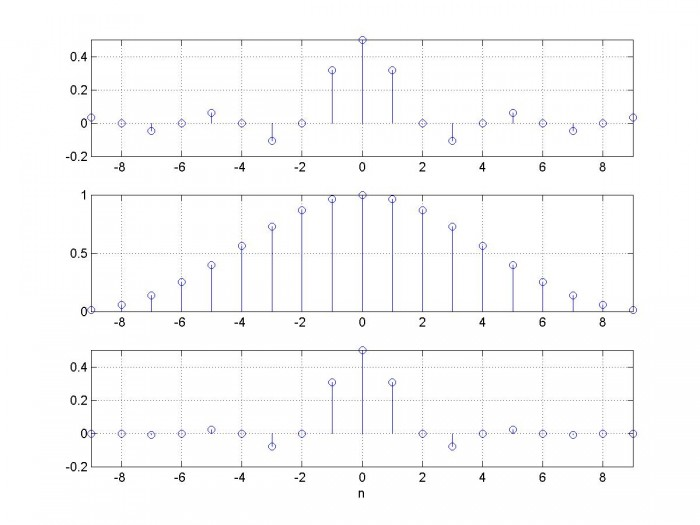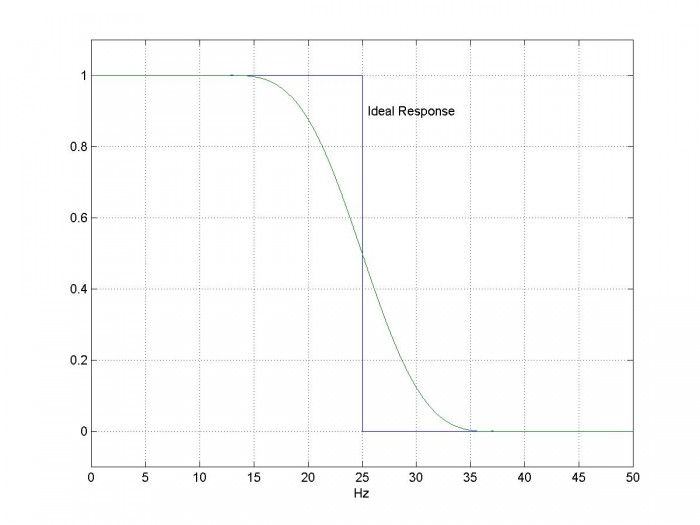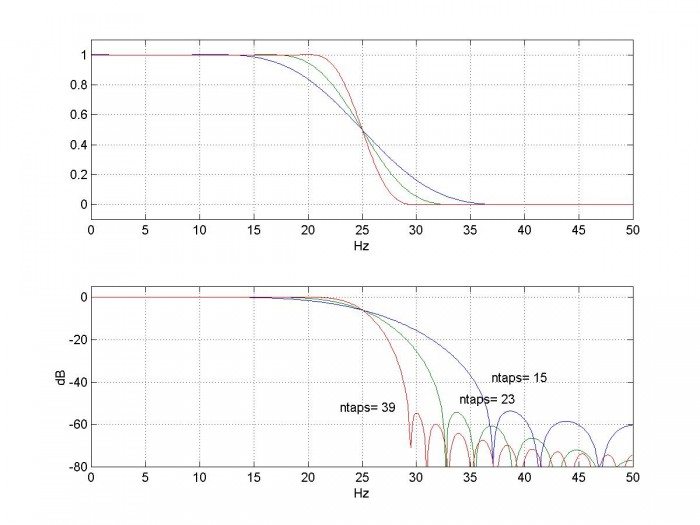# Simplest Calculation of Half-band Filter Coefficients

Half-band filters are lowpass FIR filters with cut-off frequency of one-quarter of sampling frequency fs and odd symmetry about fs/4  *.  And it so happens that almost half of the coefficients are zero.  The passband and stopband bandwiths are equal, making these filters useful for decimation-by-2 and interpolation-by-2.  Since the zero coefficients make them computationally efficient, these filters are ubiquitous in DSP systems.

Here we will compute half-band coefficients using the window method.  While the window method typically does not yield the fewest taps for a given performance, it is useful for learning about half-band filters.  Efficient equiripple half-band filters can be designed using the Matlab function firhalfband .

## Coefficients by the Window Method

The impulse response of an ideal lowpass filter with cut-off frequency ωc = 2πfc/fs is :

$$h(n)=\frac{sin(\omega_c n)}{\pi n}, \quad -\infty < n < \infty$$

This is the familiar sinx/x or sinc function (scaled by ωc/π).  To create a filter using the window method, we truncate h(n) to N+1 samples and then apply a window.  For a halfband filter, ωc = 2π*1/4 = π/2.  So the truncated version of h(n) is:

$$h(n)=\frac{sin(n\pi /2)}{n\pi}, \quad -N/2 < n < N/2$$

Now apply a window function w(n) of length N+1 to obtain the filter coefficients b:

$$b(n)=h(n)w(n), \quad -N/2 < n < N/2$$

$$b(n)=\frac{sin(n\pi /2)}{n\pi}w(n), \quad -N/2 < n < N/2 \qquad (1)$$

Choice of a particular window function w(n) is based on a compromise between transition bandwidth and stopband attenuation.  As a simple example, the Hamming window function of length N+1 is**:

$$w(n)= 0.54+0.46cos(\frac{2\pi n}{N}),\quad -N/2 < n < N/2$$

And that’s it!  Equation 1 is all you need to compute the coefficients.  Note that N is even and the filter length is N+1 (odd length).

## Examples using Matlab

Here is Matlab code that implements Equation 1 for ntaps = 19:

ntaps= 19;
N= ntaps-1;
n= -N/2:N/2;
sinc= sin(n*pi/2)./(n*pi+eps);      % truncated impulse response; eps= 2E-16
sinc(N/2 +1)= 1/2;                  % value for n --> 0
win= kaiser(ntaps,6);               % window function
b= sinc.*win';


I used the Matlab Kaiser window function b= kaiser(ntaps,beta), where beta determines the sidelobe attenuation in the frequency domain .  Higher beta gives better stopband attenuation in the halfband filter, at the expense of wider transition band.  Figure 1 shows the truncated sinc function, the window function, and the coefficients.  Every other coefficient is zero, except for the main tap.  This follows from the fact that every other sample of the truncated sinc function is zero.  The scale of the plot obscures the values of the end coefficients; b(-9) and b(9) are equal to .000526.

Figure 2 shows the magnitude response for fs= 100 Hz, with a linear amplitude scale.  The response of the ideal lowpass filter is also shown.  The amplitude is 0.5 at fs/4 = 25 Hz.  Note the odd symmetry with respect to (x,y) = (25, 0.5).

As already mentioned, ntaps must be odd.  It is possible to create a halfband frequency response with ntaps even, but in that case, there will be no zero-valued coefficients.  Also, for ntaps = 9, 13, … etc, the end coefficients are zero.  For this reason, we should choose ntaps = 4m + 3, where m is an integer.  Thus the useful values of ntaps are 7, 11, 15, etc.  The number of zero coefficients is ½(ntaps-3).

Now let’s look at the response for different values of ntaps (Figure 3).  The upper graph uses a linear amplitude scale, while the lower one uses dB.  As you can see, the stopband attenuation does not change much vs. ntaps, but the transition band becomes sharper as ntaps is increased.  The trade-off between attenuation and transition band can be changed by adjusting the Kaiser window’s beta value.

### Footnotes

*  Mathematically, $H(e^{jω}) + H(e^{j(π-ω)}) = 1$ , where ω = 2πf/fs.

**  If we let n = 0:N instead of –N/2:N/2, the expression for the Hamming window is :

$$w(n)= 0.54-0.46cos(\frac{2\pi n}{N}),\quad n= 0:N$$Figure 1.  Coefficients of Halfband Filter with ntaps = 19

top:  Truncated sinc function     center:  Kaiser window with beta = 6     bottom:  Filter coefficientsFigure 2.  Magnitude response of halfband filter with ntaps = 19, fs= 100 Hz (linear amplitude scale)Figure 3.  Halfband filter magnitude response for different length filters, fs = 100 Hz

top:  linear amplitude scale             bottom:  dB amplitude scale

## Other DSPRelated.com Posts on Halfband filters

Here are a couple of other posts about halfband filters on DSPRelated.com:

Rick Lyons, “Optimizing the Half-band Filters in Multistage Decimation and Interpolation”, Jan 2016.  https://www.dsprelated.com/showarticle/903.php

Christopher Felton, “Halfband Filter Design with Python/Scipy, Feb 2012. https://www.dsprelated.com/showcode/270.php

## References

1.  Sanjit K. Mitra, Digital Signal Processing, 2nd Ed., McGraw-Hill, 2001, p 701-702.

2.  Mathworks website https://www.mathworks.com/help/dsp/ref/firhalfband.html

3.  Mitra, p 448.

4.  Mathworks website https://www.mathworks.com/help/signal/ref/hamming.html

5.  Mathworks website https://www.mathworks.com/help/signal/ref/kaiser.html

Neil Robertson   November, 2017

## Appendix  A   Matlab Function for Halfband Filter Coefficients

This program is provided as-is without any guarantees or warranty.  The author is not responsible for any damage or losses of any kind caused by the use or misuse of the program.

%hbsynth.m       11/13/17 Neil Robertson
% Halfband filter synthesis by the window method, using a Kaiser window.
% input argument ntaps must be odd.
function b= hbsynth(ntaps)
if mod(ntaps,2)==0
error('  ntaps must be odd')
end
N= ntaps-1;
n= -N/2:N/2;
sinc= sin(n*pi/2)./(n*pi+eps);      % truncated impulse response; eps= 2E-16
sinc(N/2 +1)= 1/2;                  % value for n --> 0
win= kaiser(ntaps,6);               % window function
b= sinc.*win';                      % apply window function


## Appendix B   Matlab Code to Create Figure 3

%hb_ex1.m    11/13/17 Neil Robertson
fs= 100;
b1= hbsynth(15);
b2= hbsynth(23);
b3= hbsynth(39);
[h1,f]= freqz(b1,1,512,fs);
H1= 20*log10(abs(h1));
[h2,f]= freqz(b2,1,512,fs);
H2= 20*log10(abs(h2));
[h3,f]= freqz(b3,1,512,fs);
H3= 20*log10(abs(h3));
subplot(211),plot(f,abs(h1),f,abs(h2),f,abs(h3)),grid
axis([0 fs/2 -.1 1.1]),xlabel('Hz')
subplot(212),plot(f,H1,f,H2,f,H3),grid
axis([0 fs/2 -80 5]),xlabel('Hz'),ylabel('dB')
text(37,-38,'ntaps= 15')
text(34,-48,'ntaps= 23')
text(23,-52,'ntaps= 39')


[ - ]
Comment by November 16, 2018Hi Neil,

great article ! Do you think that a half band filter designed with this method is suitable for oversampling decimation purposes (low pass filtering oversampled signal).

Thank you !

Andreas

[ - ]
Comment by November 16, 2018Andreas,

Yes, it is appropriate.  For example, you could use a single HB filter for decimation by 2, two HB's for decimation by 4, etc.  Note in the case of decimation by 4, 8, etc., the stopband frequency range of each stage is different, so the HB filters are not normally identical to each other. See article by Rick Lyons: https://www.dsprelated.com/showarticle/903.php

For decimation by a large factor, a CIC filter may be more appropriate.

regards,

Neil

[ - ]
Comment by November 16, 2018Andreas,

I should also mention again that the window method does not typically result in the most efficient design; a Parks- McClellan optimizer like Matlab's firhalfband or firpm would be desirable for getting fewest taps.

regards,

Neil

[ - ]
Comment by November 16, 2018Fantasic, maybe the algorithm is not the most efficient, but it's easy to implement. The other article is also great, obviously the first (downsampling) filter doesn't need many taps in the fir, the last one needs. There are other approaches e.g. overlap/add in the frequency domain. I will give this easy sinc filter a try and will have a look what the performance drop of my synth plug-in is.

This is also very interesting:

[ - ]
Comment by November 18, 2018Neil, I have implemented the algorithm into my synth plug-in using C++, optimized it and tested a lot of FIR tap sizes using the great online designer here: https://fiiir.com/ and looking at the frequency response of my software at different sampling rates. I'm now using 7, 11 and 15 taps (in case of 8 x downsampling) and it seems that this is enough for my purposes. It filters the most portions of a) generated gaussion noise aliasing and b) distortion/overdrive effect aliasing. I come to the conclusion that I have a performance drop of 6-10% in comparison to the other synth functionality. For what it does, that's a moderate value but I'm thinking about additional optimizations. Another remark is, that every second tap value should really be 0 (instead of very very small float/double values). Modern CPUs seem to automatically "skip" multiplications by 0., my performance profiling showed that.

[ - ]
Comment by November 18, 2018I should add that the 6-10% performance drop was measured with an easy synth patch, with more complex patches and more polyphonic voices, the drop is about 2-3%.

[ - ]
Comment by November 18, 2018Andreas,

Good work!  Thanks for the link.

regards,

Neil

[ - ]
Comment by September 11, 2019Very intuitive and good tutorial. Thanks very much.

[ - ]
Comment by September 11, 2019You're welcome!

To post reply to a comment, click on the 'reply' button attached to each comment. To post a new comment (not a reply to a comment) check out the 'Write a Comment' tab at the top of the comments.